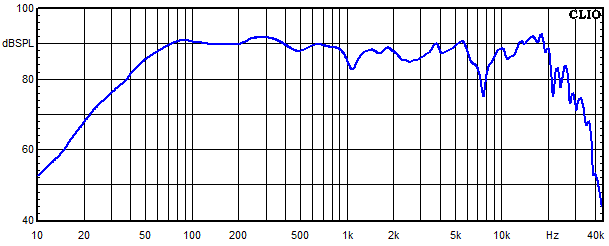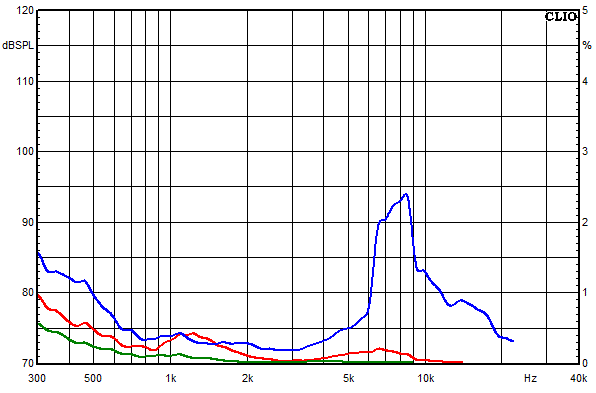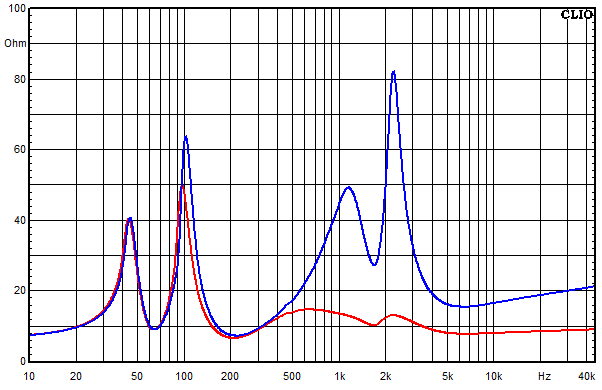Measurements TyneHere you can find the measurements for the kit Tyne.

The measurements are made with the Clio FW measurement system from Audiomatica

The measuring amplifier is a Clio QC-Box 5. This control unit performs a variety of tasks, e.g. controlling a laser, controlling turntables, etc.

For the measurements a calibrated Audiomatica Mic-01 with a length of 25 cm is used.

Frequency responseFrequency response measured at 0°, 15°, 30° and 45° angle• Blue = 0°
• Green = 15°
• Red = 30°
• Violet = 45°

Frequency response of the individual paths (for each driver)• Blue = Sum of tweeter and woofer measured on axis
• Green = high frequency branch
• Red = low frequency branch

Waterfall spectrumDistortion over frequency at 85 dB sound pressure level• Blue = k2
• Red = k3
• Green = k5

Distortion over frequency at 95 dB sound pressure level• Blue = k2
• Red = k3
• Green = k5

Impedance frequency response• Blue = without impedance correction
• Red = with impedance correction

Frequency response of the tweeter• Blue = with crossover
• Orange = without crossover

Frequency response of the woofer• Blue = with crossover
• Orange = without crossover